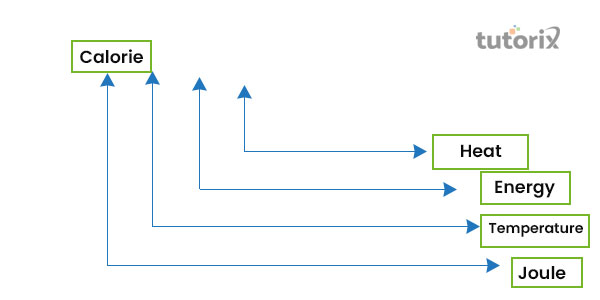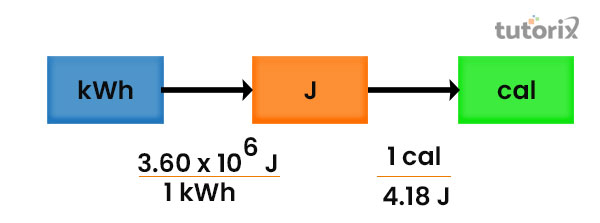# Relation between Calorie and Joule

## Introduction

Calorie and Joule are one kind of them. It is proved that when the rate of calories is increased then the value of the joules is increased with a growth rate of 4.2. On the other hand, in case of the value of the calorie decreases then the value of the joule increases at the rate of 4.2 approximately. The unit of work is equal to 107 degrees and it is equivalent to approximately 107 degrees, which are the C.G.S units of the system. The measurement of Joule is similar to the watt-second, which refers to the energy that is transferred to a particle in 1N force that is displacement by 1 meter by 1 meter in the force direction.

## What is the Relation between Calorie and Joule?

In the international SI unit three different factors like energy, work as well as heat. Joule is mostly used as the SI unit of heat that is represented by “J”.

The relation of a joule is similar to the watt, which is the power of duration that can be calculated in a second. While calorie denotes the units of energy that are more than 1000 times larger than the gram of calories. A calorie is also equivalent to the 4.2 kilojoules approximately.

### The relation between Calorie and JouleFigure 1: Measurement of Calorie and Joule

1 calorie is the amount of heat required at the pressure of in 1 standard atmosphere for raising the temperature of the 1 gram of water through 10C. The inter-relationship is the joule and calorie are both connected with the heat which can be defined variously (Uddin et al. 2021). The relationship between Calorie and Joule can be calculated with a simple formula that is 1 Calorie = 4.185 joule approximately (Feng et al. 2020).

Energy is the main component of the working ability and heat is the most significant form of energy. Heat and energy are the most important components of physics that are calculated with different units.

### The formula of Calorie and Joule

Calorie and joule are the most used physical quantities in physics that are used to calculate the heat and energy of a particle. The calculative formula between these two units is related to several purposes (Richmond, 2021). 20 calories are equal to nearly 83.68 J and 100 cal is equal to approximately 408.5 J.

In order to calculate 1 kcal one has to be calculated with 4184 J and in the same way, the 5 kcal can be calculated with 20920 J. In order to measure the energy of food, a calorie is mainly used instead of Joule. The energy of the electric power is also calculated with Joule instead of calories (Hussein & Ahmed 2021).

1 joule is equivalent to an exert of 1 Newton for and the distance of about 1 meter. 1 joule is nearly similar to a watt of 1 second of power.

## Uses of Calorie and Joule

The application of these two units is seen in several fields in the field of nutritional sciences the use of calories and joule is mostly seen. A larger calorie is commonly called a kilocalorie (Richmond, 2021). In order to measure the food value and the energy of the animal, the body is commonly calculated in kilocalories but in some places like in the US, kilojoules are used to measure the food value and the energy. The macronutrients like carbohydrates, proteins as well as fats in food particles contain about 4 kilocalories/gram while protein comprises 4 kilocalories/gram and fat 9 kilocalories/gram (Hussein & Ahmed 2021).Figure 2: The relation between Calorie and Joule

1 joule represents different scientific measurements like the power of electricity that is calculated by 1 m/s2 with the acceleration of 1 kg mass. In the property of energy calculation, particularly in the heat energy joule is used.

## Conclusion

In the current situation, calories and joule are mostly applicable to measure the energy and the heat of different particles whether in physics or chemical fields. In the calculation of the human energy joule and calories, both units are used with the formula that each 1/60 s which is approximately 17ms is equivalent to 1 joule. The energy and the temperature of the body particles are also calculated by the calorie and joule. In terms of measuring the water, solvent calories are also used. In the field of experimental calorimetry, these two units are aslo seen severally.

## FAQs

Q.1 What is the difference between calorie and joule?

Both units are used to calculate the energy, heat and power of the body particles. Most of cases the nutrition value of food and the human body's energy are calculated with calories while the temperature and the chemical energy are calculated through joule.

Q.2. What is the formula of calorie and joule?

The basic formula of calorie and joule is 1 cal is equivalent to approx 4.184 J. For preference, 4.184 is considered as 4.2 J.

Q.3. What is the application of calorie and joule?

In the chemical field, the use of calories and joule is also commonly seen. In order to measure the energy that releases during the chemical reaction, the calorie and joule are commonly used. Besides this, in biological prospects, these two units are also severally used.

Q.4 What is the process of converting calories to joule?

The initial process of converting calorie top joule is 1 cal = about 4.2 J. In a larger concept, 1 kcal = 4184 J. This formula is the easiest way to convert calories to a joule.

## References

### Journals

Feng, Z. W., Zhou, X., He, G., & Zhou, S. Q. (2020). Joule-Thomson expansion of higher dimensional nonlinearly charged AdS black hole in Einstein-PMI gravity. arXiv preprint arXiv:2009.02172. Retrieved from: https://arxiv.org/pdf/2009.02172

Hussein, I. S., & Ahmed, B. A. (2021). CALCULATED CALORIES FOR TRAINING AND THEIR EFFECT ON DEVELOPING SOME PHYSICAL AND BIOMECHANICAL ABILITIES AND ACHIEVING A 400-METER RUN FOR YOUTH. European Journal of Physical Education and Sport Science, 6(10). Retrieved from: https://www.oapub.org/edu/index.php/ejep/article/download/3589/6224

Minamitani, E. (2021). Ab initio analysis for the initial process of Joule heating in semiconductors. Physical Review B, 104(8), 085202. Retrieved from: ( https://arxiv.org/pdf/2107.03553

Richmond, A. D. (2021). Joule heating in the thermosphere. Upper Atmosphere Dynamics and Energetics, 1-18. Retrieved from: https://cedarscience.org/sites/default/files/workshops/2022/Richmond2020preprint.pdf

Uddin, I., Ullah, I., Ali, R., Khan, I., & Nisar, K. S. (2021). Numerical analysis of nonlinear mixed convective MHD chemically reacting flow of Prandtl–Eyring nanofluids in the presence of activation energy and Joule heating. Journal of Thermal Analysis and Calorimetry, 145(2), 495-505. Retrieved from: https://www.researchgate.net/profile/Ali-8/publication/341411314_Numerical_analysis_of_nonlinear_mixed_convective_MHD_chemically_reacting_flow_of_Prandtl-Eyring_nanofluids_in_the_presence_of_activation_energy_and_Joule_heating/links/5ecd71ee92851c9c5e5f490c/Numerical-analysis-of-nonlinear-mixed-convective-MHD-chemically-reacting-flow-of-Prandtl-Eyring-nanofluids-in-the-presence-of-activation-energy-and-Joule-heating.pdf

Updated on: 21-Aug-2023

548 Views